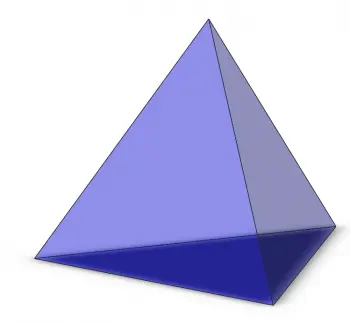# Triangular Pyramid: Volume, Faces, Vertices and EdgesThe triangular pyramids are pyramids with a triangular base, polyhedrons whose faces are formed by triangles.

In this article, we explore the geometry behind the triangular-based pyramids and how it is applied in the construction of these impressive structures.

## Characteristics of Triangular Base Pyramids

1. Number of edges: 6. Triangular pyramids have a total of six edges, which are the straight edges that connect the vertices of the base with the vertex of the tip. Each edge is formed by two sides of adjacent triangles.

2. Number of faces: 4. Triangular pyramids have a total of four triangular faces, including the base and three lateral faces.

3. Number of vertices: 4. These geometric bodies have four vertices: the three vertices of the base and the vertex of the tip. The vertex of the tip is the point where the three lateral faces of the pyramid meet, and the three vertices of the base are the points where the sides of the base triangle meet.

4. Height: The height is the distance from the common vertex to the base. The height of the pyramid is perpendicular to the base and is located in the center of it.

5. Symmetry: Regular triangular-based pyramids have rotational symmetry of 120 degrees about a vertical axis through the common vertex and the center of the base. This means that the pyramid looks the same from any angle of rotation of 120 degrees around this axis.

6. Special Properties: Pyramids with a triangular base have a number of interesting mathematical properties, including the fact that their height is always inside the pyramid and is less than half the length of the base.

## Volume of a Triangular Pyramid: Formula and Example

To calculate the volume of a triangular pyramid, you need to know the measurement of the height and the length of the base of the pyramid. The calculation of the volume is made using the following formula:

V = (B x h) / 3

Where:

• V is the volume of the pyramid.

• B is the area of ​​the base.

• h is the height.

To calculate the area of ​​the base, the formula for calculating the area of ​​a triangle is used:

B = (b x a) / 2

Where:

• B is the area of ​​the base.

• b is the length of one of the sides of the base of the triangle.

• a is the height of the triangle

Once the area of ​​the base is known, the above formula can be used to find the volume of the pyramid.

### Volume Calculation Example

Suppose we have a pyramid with a triangular base with a height of 10 cm and a base of length 8 cm. To calculate the volume of the pyramid, you must first calculate the area of ​​the base:

B = (b x a) / 2

B = (8cm x 8cm) / 2

B = 32cm²

Once the area of ​​the base is known, the volume of the pyramid can be calculated:

V = (B x h) / 3

V = (32 cm² x 10 cm) / 3

V = 320 cm³ / 3

V = 106.67 cm³

Therefore, the volume of the pyramid with a triangular base is 106.67 cm³.

## Types of Triangular Pyramids

There are several types of pyramids with a triangular base that differ according to their proportions and angles. Next, we will describe some of the most common types:

1. Equilateral pyramid: all sides of the base are equal and all angles are 60 degrees. The height of the pyramid is perpendicular to the base and passes through the midpoint of each side. This is one of the simplest and most symmetrical pyramids in existence. Pyramids of this type are also known as tetrahedrons.

2. Isosceles pyramid: two sides of the base are equal and the third side is different. The angles opposite the equal sides are also equal. The height of the pyramid is perpendicular to the base and divides the base triangle into two congruent right triangles.

3. Scalene pyramid: all the sides of the base are different and all the angles are also different. The height is not perpendicular to the base and does not pass through the midpoint of each side.

4. Oblique pyramid: the base is not perpendicular to the axis of the geometric body and the height is not contained in the plane of the base.

Author:

Published: May 7, 2023
Last review: May 7, 2023